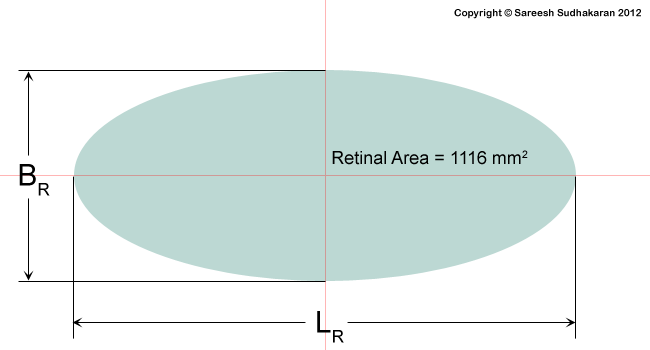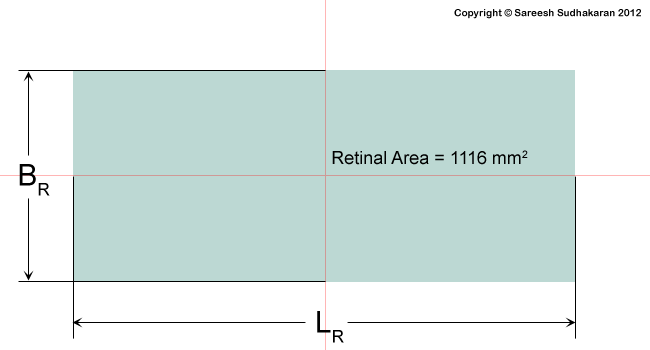Categories

# Notes by Dr. Optoglass: Angle of View and Aspect Ratio

Topics Covered:

• The Angle of View of the Eye
• The Sensor Size of the Eye
• The Aspect Ratio of the Eye
• The Crop Factor (35mm equivalent) of the Eye

The eye of the master will do more work than both his hands – Benjamin Franklin

Put together, the horizontal angle of view of both eyes is about 180o. If you rotate your eyes, you can even see behind you – but one side at a time.

However, the angle of view of each eye is about a total of 155o horizontally and 135o vertically (The Ophthalmic Assistant – Stein, Slatt, Stein pg.395).

Since cameras have rectangular image areas we are used to something called the Aspect Ratio – the ratio of length to breadth.

Assuming the optical axis is perfectly in the center, the length (L) by breadth (B) is given by the formula:where AL is the horizontal angle of view (155o) and AB is the vertical angle of view (135o).

This gives us an L/B value of 1.87, which tells us the aspect ratio of the eye is 1.87:1.

If we want to take both eyes together the aspect ratio of the eye almost resembles CinemaScope! You should keep in mind that these calculations shouldn’t be taken too seriously.

Now we can get back to mapping our retina! Here’s what it could look like in theory:Where LR is the length of the ellipse mapped by the retina and BR is its breadth.

We know our aspect ratio, so we can say LR / BR = 1.87.

The simple area of an ellipse is given by the formula Area = ? (LR/2) (BR/2) where ? is pi with a value of 3.14. We already know that the total retinal area is 1116 mm2.

From the above two equations, LR = 51.56mm and BR = 27.57mm. Our retina resembles a widescreen medium format sensor!

I’m sure you’re itching to know the crop factor of the eye when compared to 35mm film. Well, why not?

The horizontal crop factor of the eye is 36mm / 51.56mm = 0.7
The vertical crop factor of the eye is 24mm / 27.57mm = 0.87
The diagonal crop factor (the one that is used the most) is 43.27mm / 57.65mm = 0.75.

Just for fun’s sake, what if we map our retina into a rectangle, instead of an ellipse? This is what it would look like:In this case LR = 45.7mm and BR = 24.43mm. Good to know, let’s move on.

What next? Let’s calculate the focal length equivalents of the eye!

Takeaways:

• The Angle of View of the eye is 155o by 135o.
• The Aspect Ratio of the Eye is about 1.87:1.
• The dimensions of the mapped retina is 51.56mm by 25.57mm (as an ellipse).
• The approximate crop factor (in 35 equivalent terms) of the eye is about 0.75.